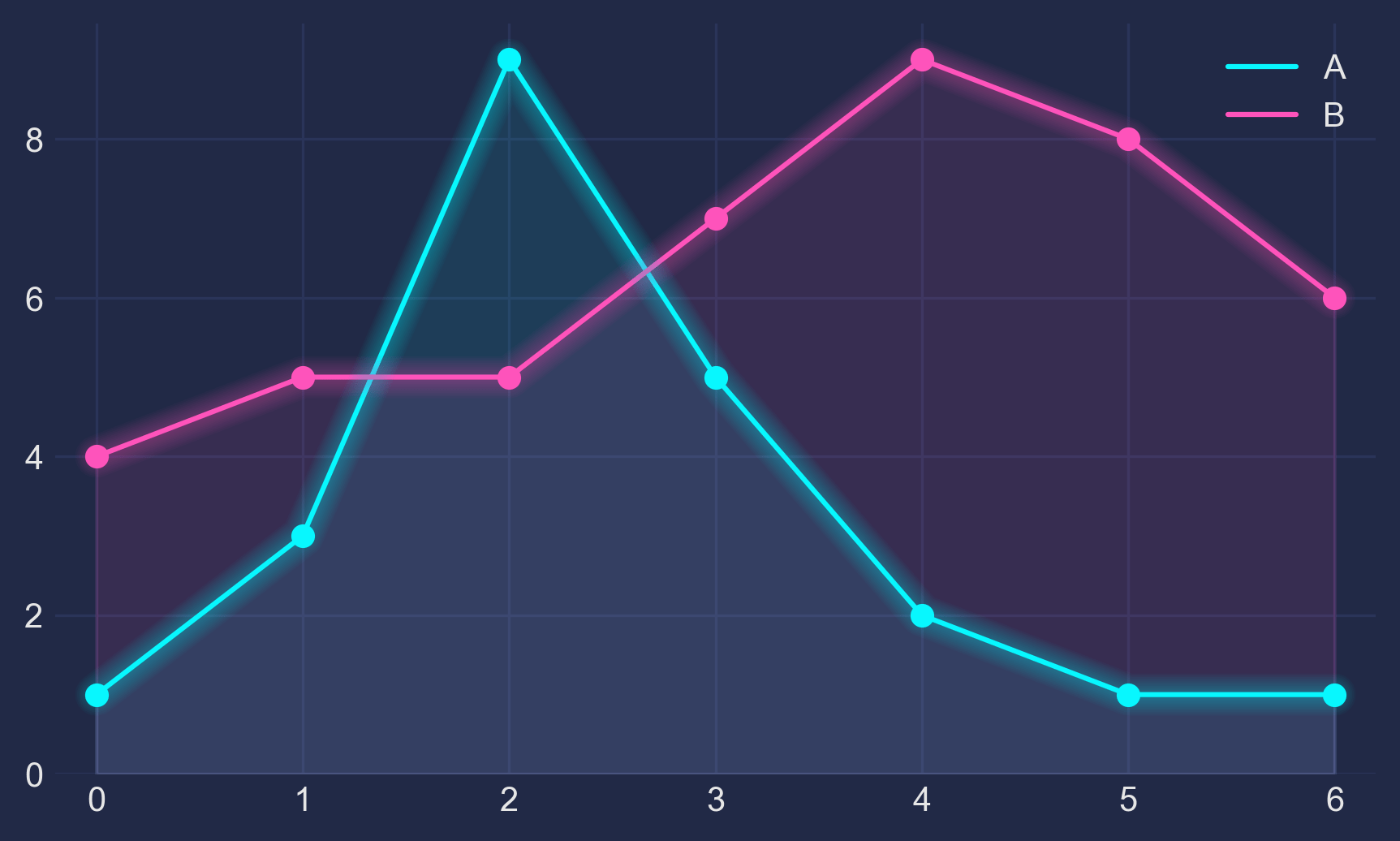## 1 - The Basis#

Let’s make up some numbers, put them in a Pandas dataframe and plot them:

``````import pandas as pd
import matplotlib.pyplot as plt

df = pd.DataFrame({'A': [1, 3, 9, 5, 2, 1, 1],
'B': [4, 5, 5, 7, 9, 8, 6]})

df.plot(marker='o')
plt.show()
``````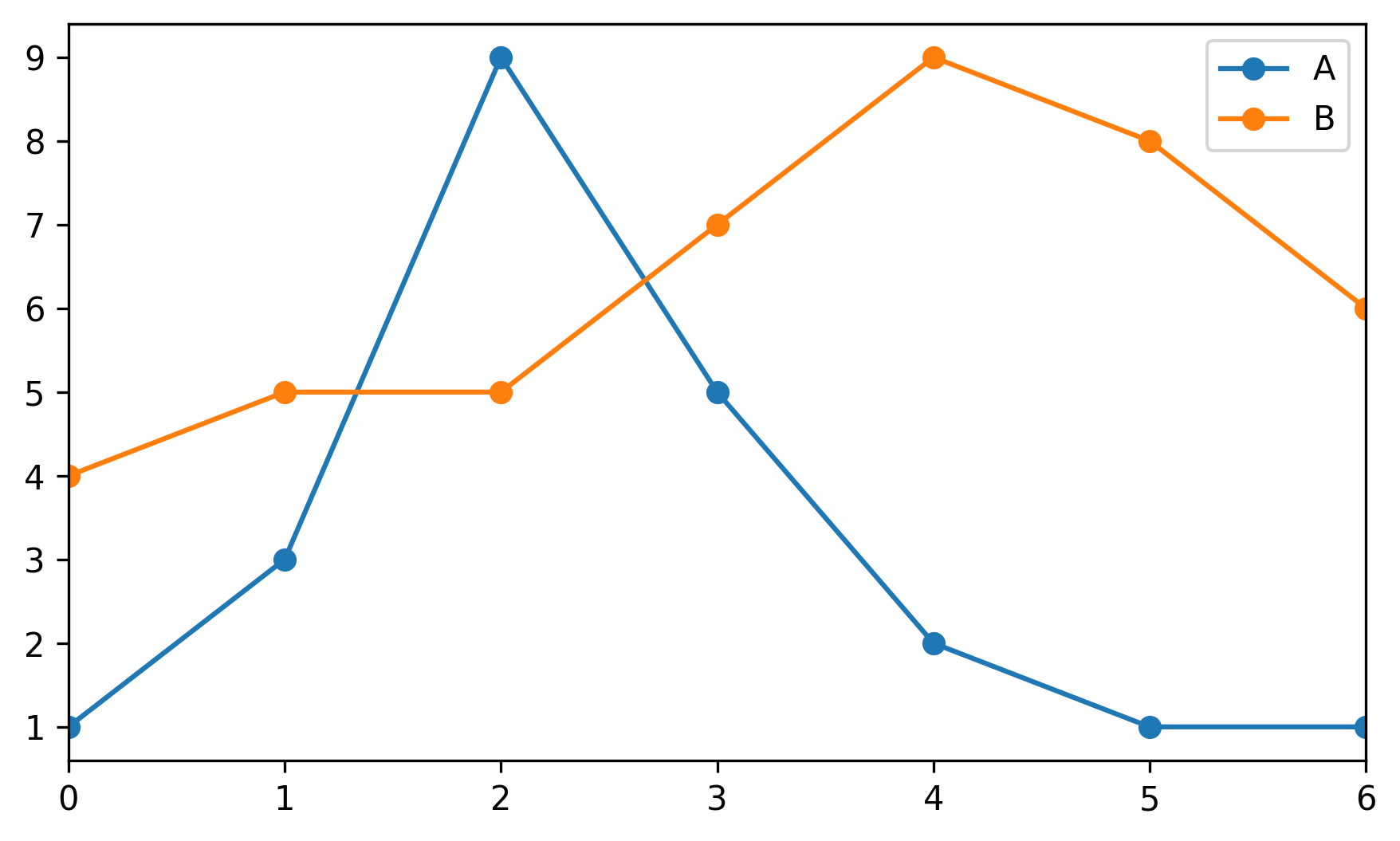## 2 - The Darkness#

Not bad, but somewhat ordinary. Let’s customize it by using Seaborn’s dark style, as well as changing background and font colors:

``````plt.style.use("seaborn-dark")

for param in ['figure.facecolor', 'axes.facecolor', 'savefig.facecolor']:
plt.rcParams[param] = '#212946'  # bluish dark grey

for param in ['text.color', 'axes.labelcolor', 'xtick.color', 'ytick.color']:
plt.rcParams[param] = '0.9'  # very light grey

ax.grid(color='#2A3459')  # bluish dark grey, but slightly lighter than background
``````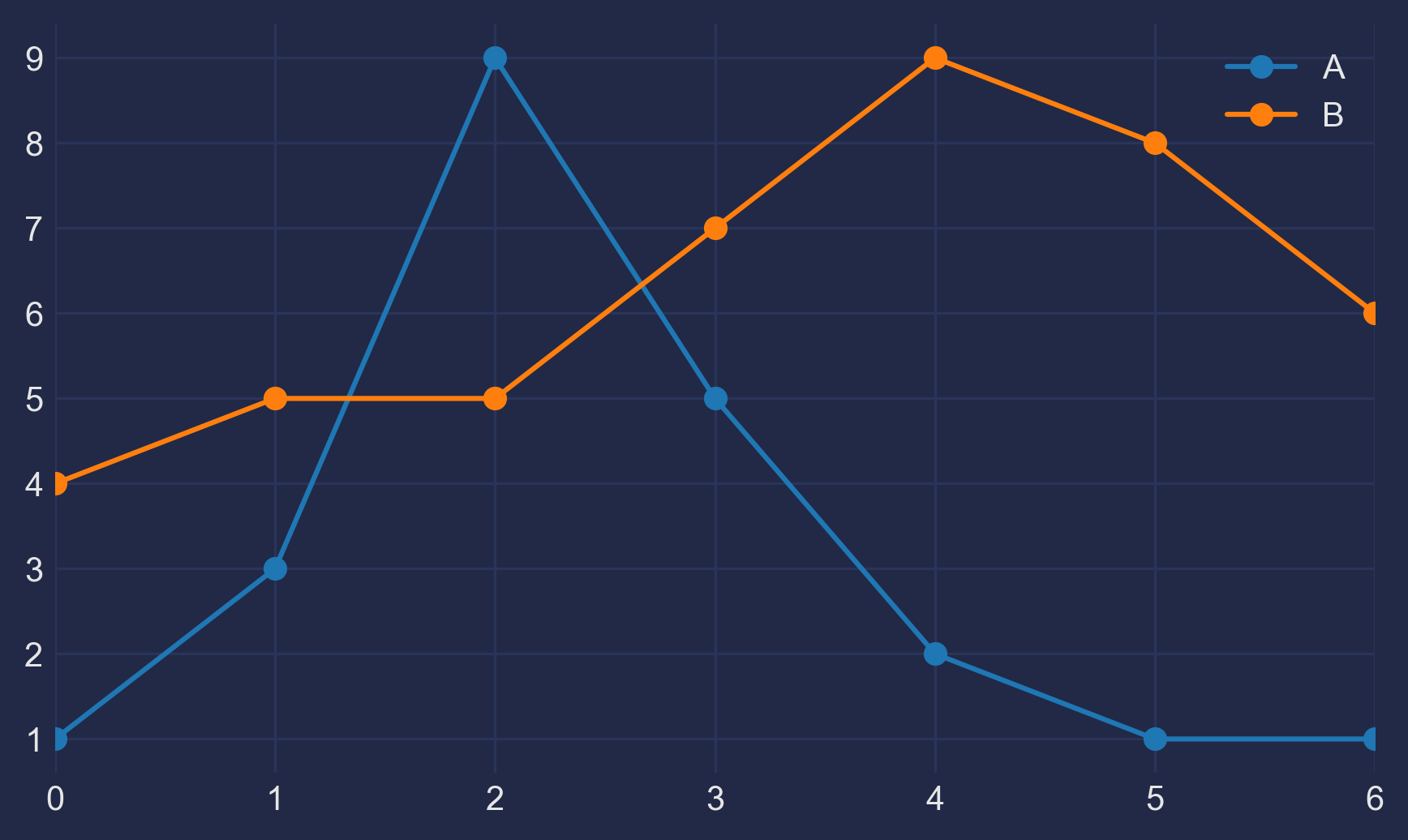## 3 - The Light#

It looks more interesting now, but we need our colors to shine more against the dark background:

``````fig, ax = plt.subplots()
colors = [
'#08F7FE',  # teal/cyan
'#FE53BB',  # pink
'#F5D300',  # yellow
'#00ff41', # matrix green
]
df.plot(marker='o', ax=ax, color=colors)
``````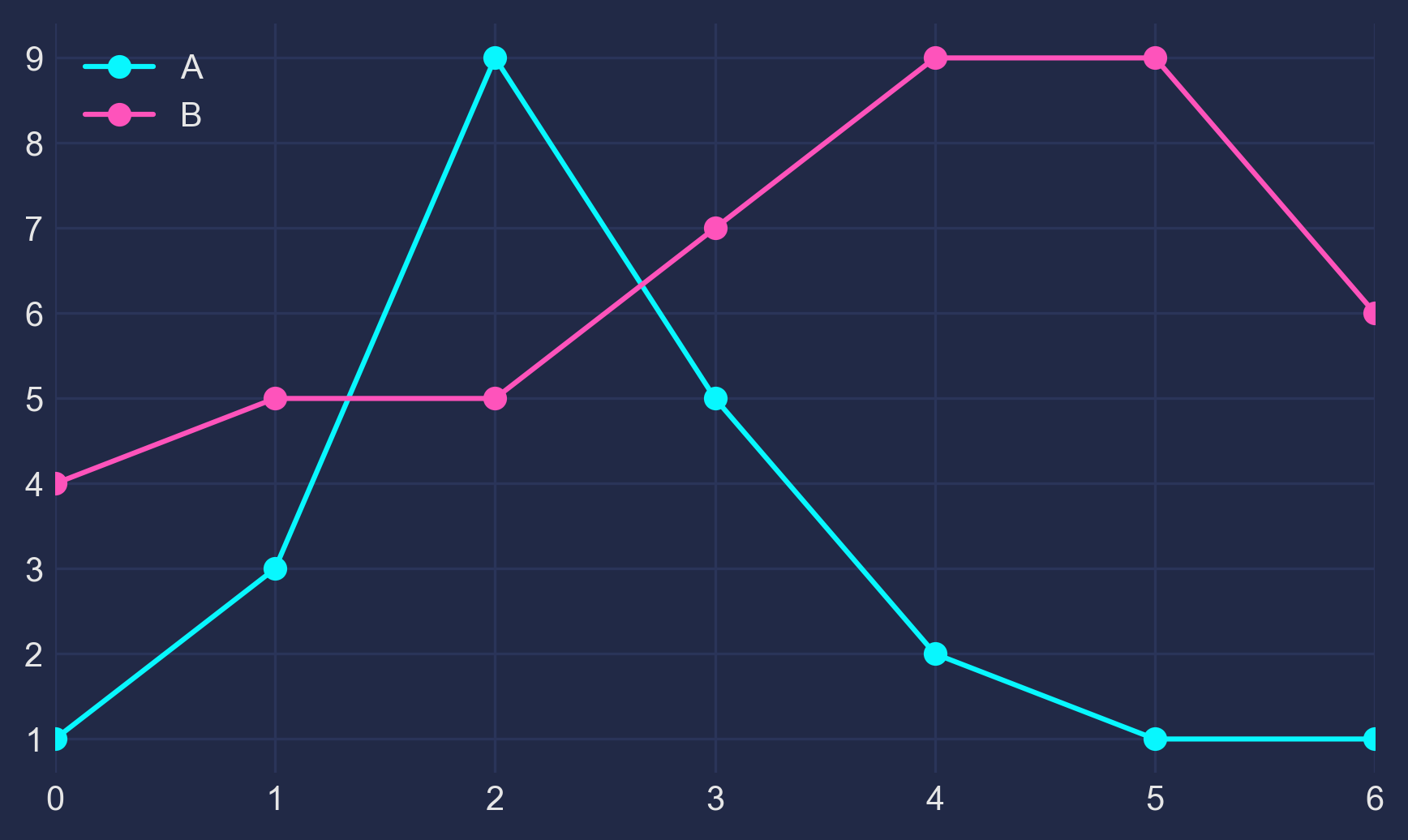## 4 - The Glow#

Now, how to get that neon look? To make it shine, we redraw the lines multiple times, with low alpha value and slightly increasing linewidth. The overlap creates the glow effect.

``````n_lines = 10
diff_linewidth = 1.05
alpha_value = 0.03

for n in range(1, n_lines+1):

df.plot(marker='o',
linewidth=2+(diff_linewidth*n),
alpha=alpha_value,
legend=False,
ax=ax,
color=colors)
``````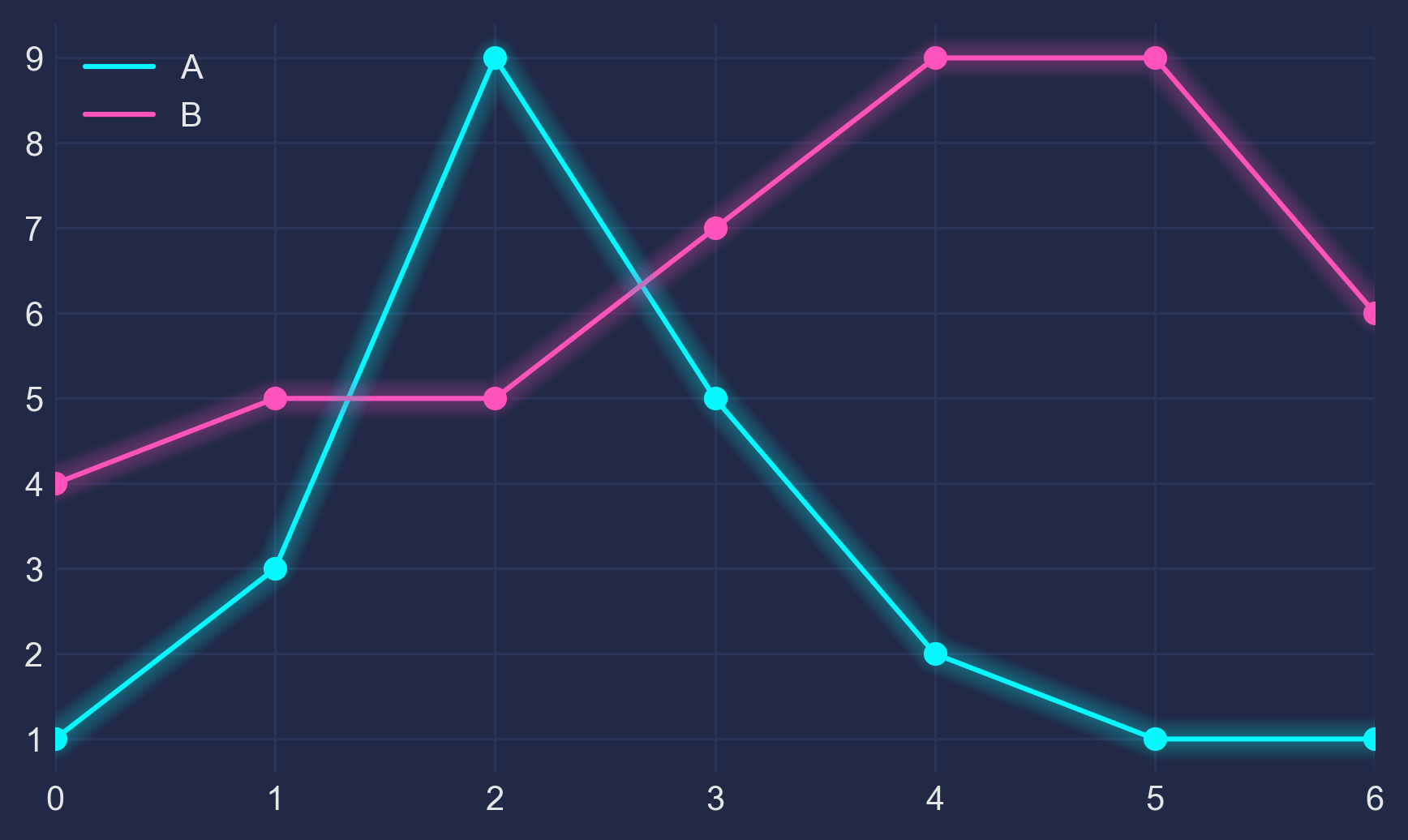## 5 - The Finish#

For some more fine tuning, we color the area below the line (via `ax.fill_between`) and adjust the axis limits.

Here’s the full code:

``````import pandas as pd
import matplotlib.pyplot as plt

plt.style.use("dark_background")

for param in ['text.color', 'axes.labelcolor', 'xtick.color', 'ytick.color']:
plt.rcParams[param] = '0.9'  # very light grey

for param in ['figure.facecolor', 'axes.facecolor', 'savefig.facecolor']:
plt.rcParams[param] = '#212946'  # bluish dark grey

colors = [
'#08F7FE',  # teal/cyan
'#FE53BB',  # pink
'#F5D300',  # yellow
'#00ff41',  # matrix green
]

df = pd.DataFrame({'A': [1, 3, 9, 5, 2, 1, 1],
'B': [4, 5, 5, 7, 9, 8, 6]})

fig, ax = plt.subplots()

df.plot(marker='o', color=colors, ax=ax)

# Redraw the data with low alpha and slightly increased linewidth:
diff_linewidth = 1.05

df.plot(marker='o',
linewidth=2+(diff_linewidth*n),
alpha=alpha_value,
legend=False,
ax=ax,
color=colors)

# Color the areas below the lines:
for column, color in zip(df, colors):
ax.fill_between(x=df.index,
y1=df[column].values,
y2= * len(df),
color=color,
alpha=0.1)

ax.grid(color='#2A3459')

ax.set_xlim([ax.get_xlim() - 0.2, ax.get_xlim() + 0.2])  # to not have the markers cut off
ax.set_ylim(0)

plt.show()
``````If this helps you or if you have constructive criticism, I’d be happy to hear about it! Please contact me via here or here. Thanks!